β

# 机器学习，Hello World from Javascript!

Taobao FED | 淘宝前端团队 43 阅读## 导语

JavaScript 适合做机器学习吗？这是一个问号。但每一位开发者都应该了解机器学习解决问题的思维和方法，并思考：它将会给我们的工作带来什么？同样，算法能力可能会是下一阶段工程师的标配。

• 你不需要具备 Python、C++ 的编程能力：全文使用 JavaScript 作为编程语言，且不依赖任何第三方库实现机器学习算法。
• 你不需要具备算法能力和高数的背景，本文机器学习算法的实现不过 20 行代码。

## 机器学习中的 Hello World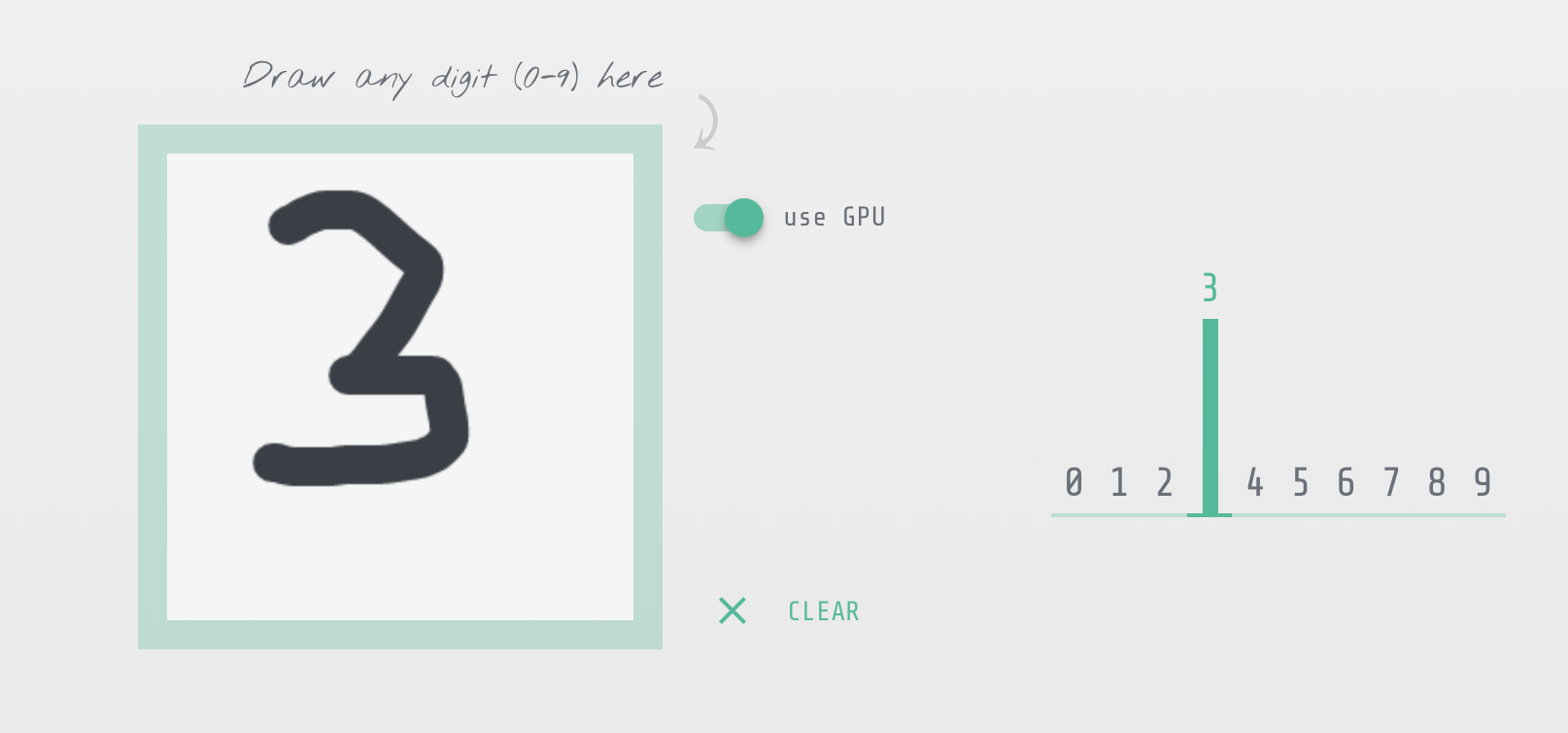### 数据的表示和收集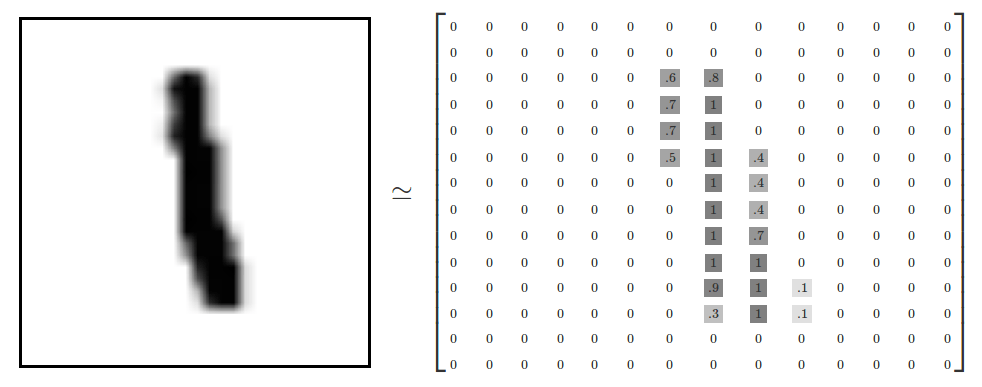`` 0.3 0 1 0 ... n(=28) | 41 0 0.1 0 0 0 ... n(=28) | 6....n(=1000)``

• 数据是一切机器学习的基础；
• 训练数据的好坏将会影响到我们机器学习算法预测的准确率：
• 想象一下如果某些数据我们标识错误，把 1 标识成 2；
• 想象一下如果训练数据中有大量的重复值，或某个数字的数据量特别大而另外一些数字的数据量很小。

### 准备数据

``[  {    input: [0, 0.4, 0.5, 0, 0.1, 0, 0, 0, 0, ..., n], // n = 728    output: [0, 1, 0, 0, 0, 0, 0, 0, 0, 0], // 1  },  {    input: [1, 0.4, 0.5, 0, 0.8, 0, 0.1, 0, 1, ..., n], // n = 728    output: [0, 0, 1, 0, 0, 0, 0, 0, 0, 0], // 2  },  ....]``

### 选择一种算法

#### k-近邻算法概述

Index Point 1 Point 2 Area
a 1 1 A
b 2 2 A
c 9 9 B
d 8 8 B
e 8.5 8.5 ?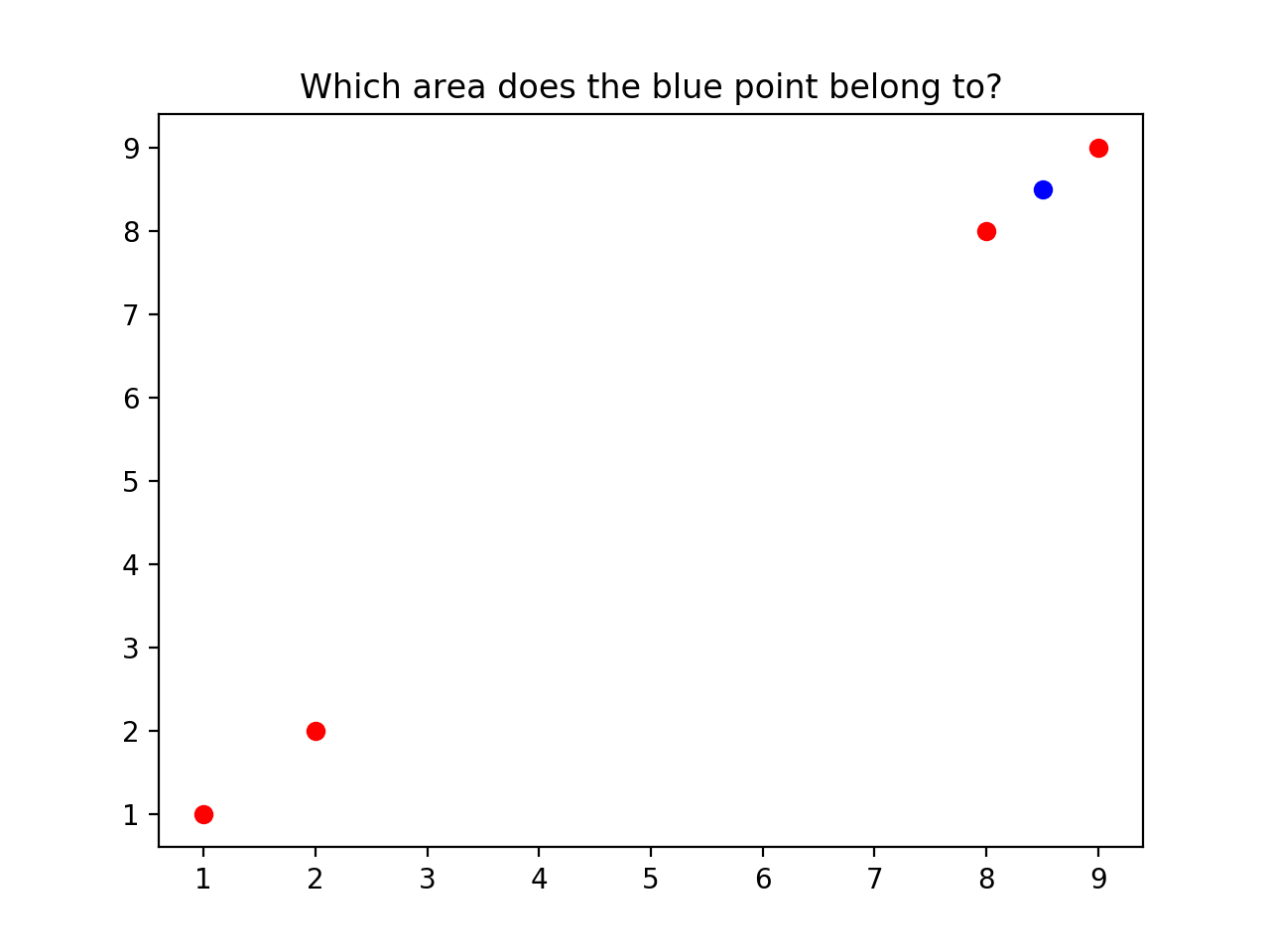——《机器学习实战》k 近邻算法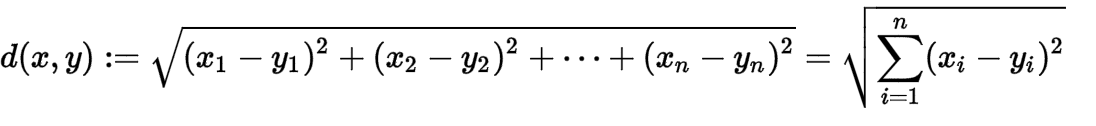``function classify(x, trainingData, labels, k) {  // 确定目标点 x 与训练数据中每个点的距离（欧几里得距离公式）  const distances =[];  trainingData.forEach(element => {    let distance = 0;    element.forEach((value, index) => {      const diff = x[index] - value;      distance += (diff * diff);    });    distances.push(Math.sqrt(distance));  });  // 将训练数据按照与 x 点的距离从近到远排序  const sortedDistIndicies = distances    .map((value, index) => {      return {value, index};    })    .sort((a, b) => a.value - b.value );  // 确定前 k 个点类别的出现频率  const classCount = {};  for (let i = 0; k > i; i++) {    const voteLabel = labels[sortedDistIndicies[i].index];    classCount[voteLabel] = (classCount[voteLabel] || 0) + 1;  }  // 返回出现频率最高的类别作为当前点的预测分类  let predictedClass = &apos;&apos;;  let topCount = 0;  for (const voteLabel in classCount) {    if (classCount[voteLabel] > topCount) {      predictedClass = voteLabel;      topCount = classCount[voteLabel];    }  }  return predictedClass;}``

#### 测试算法

``const classify = require(&apos;./kNN&apos;);// 1. 收集数据：忽略，直接使用 MNISTconst mnist = require(&apos;mnist&apos;);// 2. 准备数据let trainingImages = [];let labels = [];// 划分数据const trainingCount = 8000;const testCount = 2000;const set = mnist.set(trainingCount, testCount);const trainingSet = set.training;const testSet = set.test;// 为我们的 k-NN 算法准备特定的数据格式trainingSet.forEach(({input, output}) => {  // One-Hot to number  const number = output.indexOf(output.reduce((max, activation) => Math.max(max, activation), 0));  trainingImages.push(input);  labels.push(number);});// 3. 分析数据：在命令行中检查数据，确保它的格式符合要求console.log(&apos;trainingImages&apos;, JSON.stringify(trainingImages));console.log(&apos;labels&apos;, JSON.stringify(labels));// 4. 测试算法let errorCount = 0;const startTime = Date.now();testSet.forEach(({input, output}, key) => {  const number = output.indexOf(output.reduce((max, activation) => Math.max(max, activation), 0));  const predicted = classify(input, trainingImages, labels, 3);  const result = predicted == number;  console.log(`\${key}. number is \${number}, predicted is \${predicted}, result is \${result}`);  if (!result) {    errorCount++;  }});console.log(`The total number of errors is: \${errorCount}`);console.log(`The total error rate is: \${errorCount/testCount}`);console.log(`Spend: \${(Date.now() - startTime) / 1000}s`);``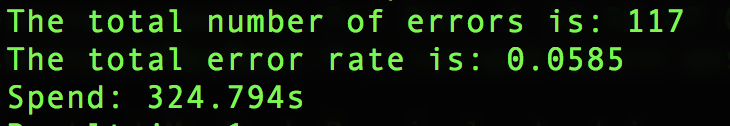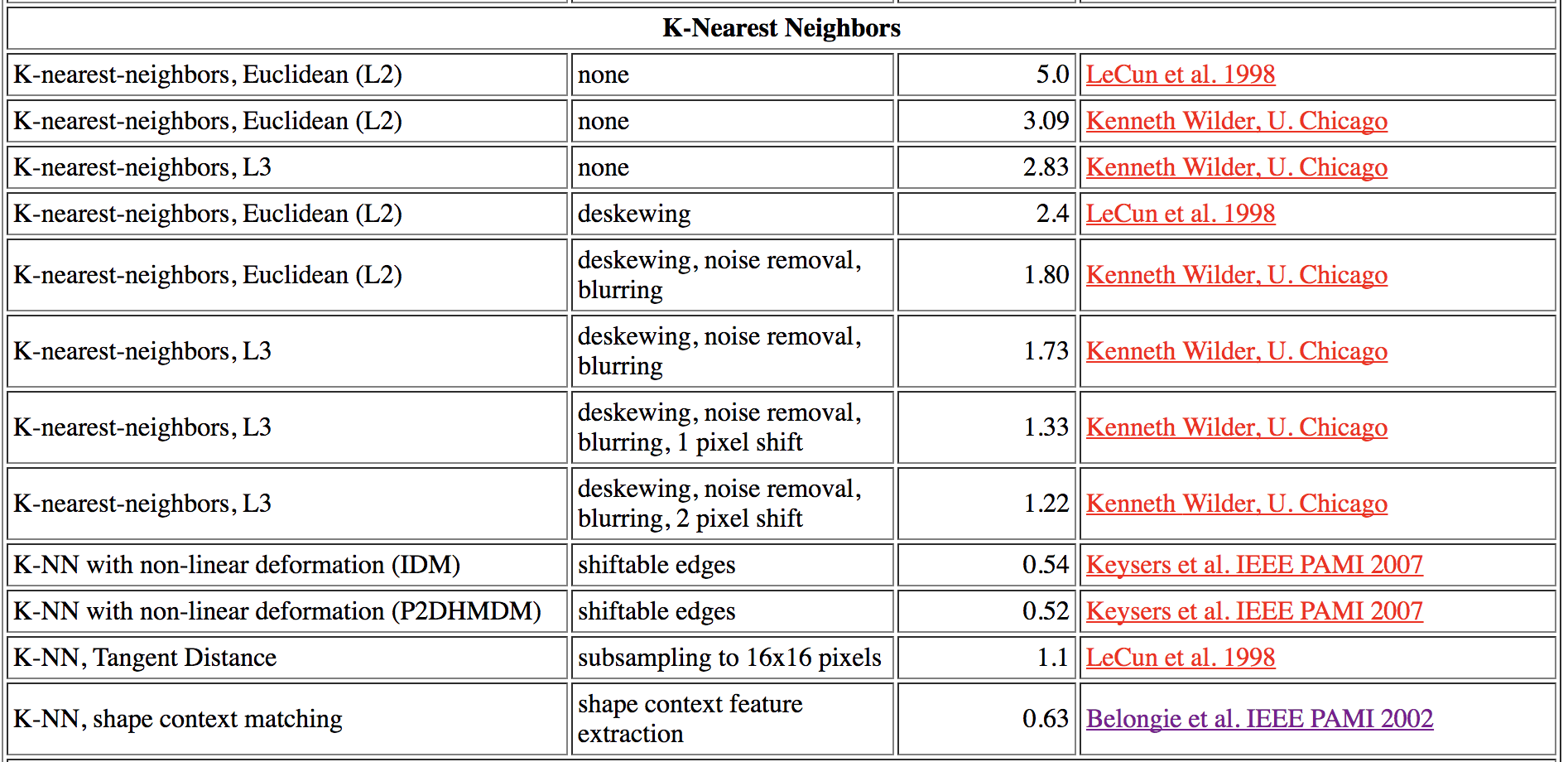#### 使用算法

``// 手写识别程序将用户输入的图像转换为我们期望的数据格式const input = [0, 0.3, 1, 1, 0, 0, 0.2, ...];// 执行算法classify(input, trainingImages, labels, 3);``

## 进一步思考

• 对于手写字，还有没有其他的数据表示方式？例如我们非要用 0 到 1 的数值来表示点的黑白度吗？
• 训练数据集是越大越好吗？例如我们将手写字所有的特征排列组合 (28^28) 个数据量作为训练数据集；
• 如何调整算法参数以获得最佳的收益（准确率和效率）？

## 参考资料